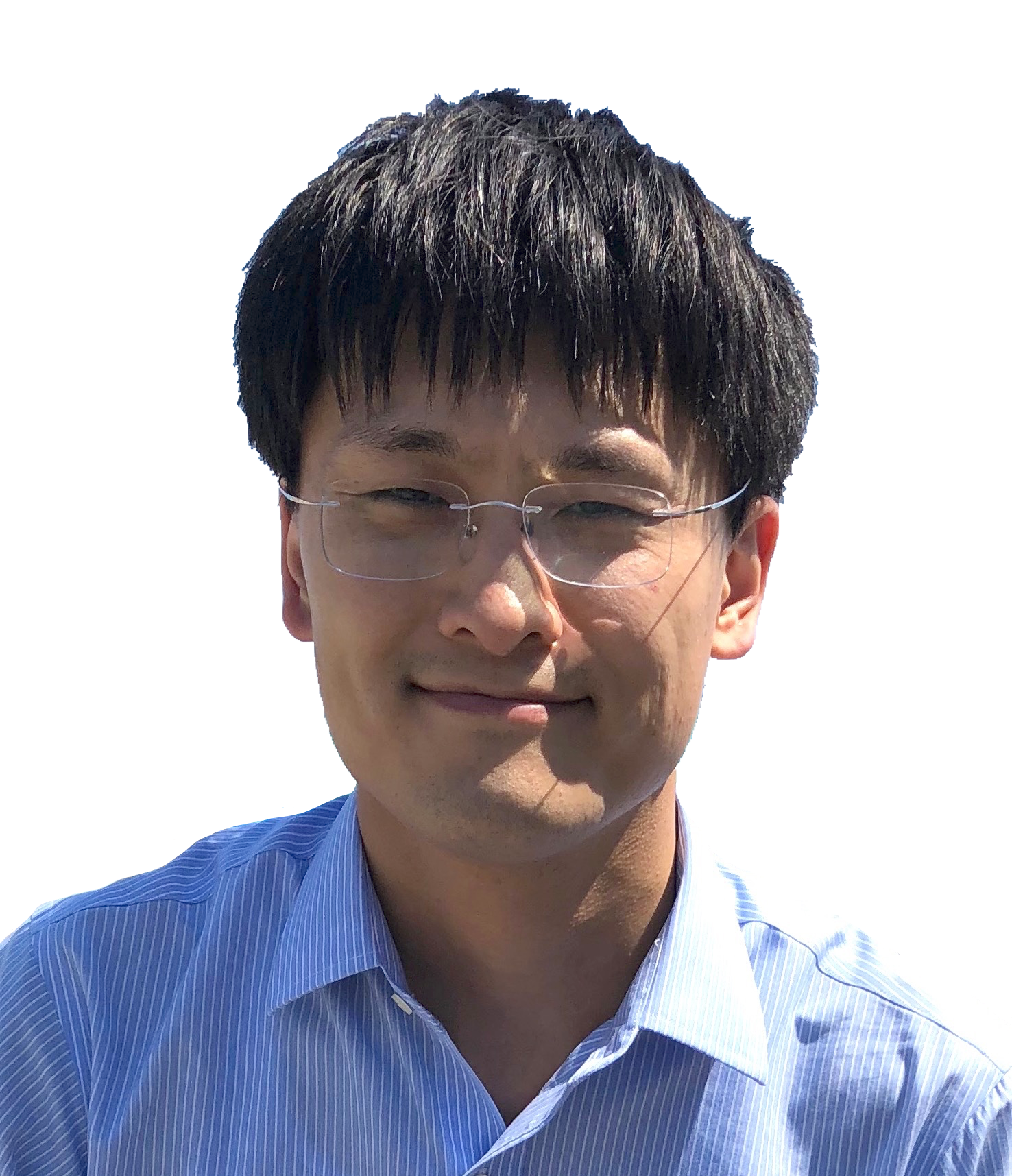DocumentProfessor and RGC Research Fellow
Department of Applied Mathematics
The Hong Kong Polytechnic University
Hung Hom, Hong Kong
https://orcid.org/0000-0001-7566-3464

### Research Interests     Publication     Research Group     PhD and Postdoctoral Positions

• Numerical methods and analysis for partial differential equations, including
• Surface evolution under geometric flows, geometric evolution equations, PDEs on surfaces ← click here
• Low-regularity approximation to nonlinear dispersive equations
• Incompressible Navier–Stokes equations
• Semilinear parabolic equations and phase field equation
• Interior penalty finite element methods and perfectly matched layer (PML) for the Helmholtz equation
• Maximal Lp-regularity of time discretization methods for parabolic equations
• Maximum-norm stability and maximal Lp-regularity of finite element methods
1. Maximum-norm stability of the finite element method for the Neumann problem in nonconvex polygons with locally refined mesh (PDF)
2. Maximal regularity of multistep fully discrete finite element methods for parabolic equations (PDF)
3. Analyticity, maximal regularity and maximum-norm stability of FEM for parabolic equations in nonconvex polyhedra (PDF)
4. Maximal Lp error analysis of FEMs for nonlinear parabolic equations with nonsmooth coefficients (PDF)
5. Maximal Lp analysis of FEM for parabolic equations with nonsmooth coefficients in convex polyhedra (PDF)
6. Maximum-norm stability and maximal Lp regularity of FEMs for parabolic equations with Lipschitz continuous coefficients (PDF)
• High-order approximation of singular solutions of fractional evolution equations
• Dynamic Ginzburg–Landau superconductivity equations in nonsmooth domains
• Time-dependent Joule heating problem (for thermistors with temperature-dependent electric conductivity (PDF)
• ### Editorial boards

• Mathematics of Computation:                 2022.2 –– present

• SIAM Journal on Numerical Analysis:   2022.1 –– present

### Awards

• RGC Research Fellow Award 2023 (by the Hong Kong Research Grants Council).

• The Hong Kong Mathematical Society Young Scholars Award 2022.

• Alexander von Humboldt Research Fellowship, 2015-2016.

• Best Paper Award for Young Scholars 2013, The Chinese Society for Computational Mathematics
(于第十二届全国高校计算数学年会).